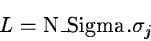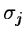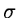Next: COMPAR/WAVE Up: Commands Description Previous: VISUAL/PERS

### FILTER/WAVE

FILTER/WAVE I_In I_Out [Algo] [T_Filter] [Iter_Nbr] [N_Scale] [N_Sigma] [Noise]
Filters an image in the wavelet space (see section 14.6). The used algorithm for the transform depends on the parameter Algo (see TRANSF/WAVE). T_Filter defines the type of filtering. It can take the values 1,2,3,4 (default value is 1):
1.
Thresholding (see section 14.6.4).
2.
Hierarchical Thresholding (see section 14.6.5).
3.
Hierarchical Wiener Filtering (see section 14.6.3).
4.
Multiresolution Wiener Filtering (see section 14.6.2).
If we threshold (T_Filter = 1 or 2), the reconstruction can be done iteratively, and the parameter Iter_Nbr specifies the number of iterations (by default it is 1, no iteration). N_Scale is the number of scales (default value is 4). N_Sigma is used only if we threshold. We consider that at a given scale j, the significant level L is:whereis the standard deviation of the noise at the scale j. Noise is the standard deviation of the noise in the image. If Noise equal to 0 (0 is the default value), the standard deviation is estimated automatically in the program from the histogram of the image by a 3clipping.Next: COMPAR/WAVE Up: Commands Description Previous: VISUAL/PERS
Petra Nass
1999-06-15